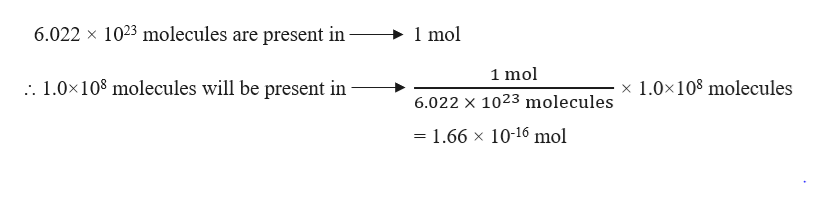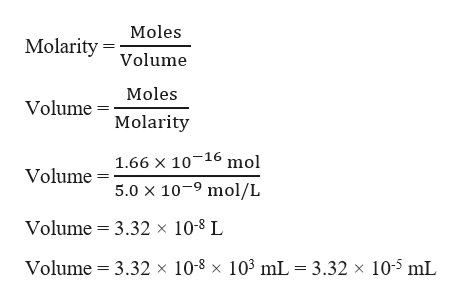# Calicheamicin gamma-1, C55H74IN3O21S4, is one of the most potent antibiotics known: One molecule kills one bacterial cell.Describe how you would (carefully!) prepare 25.00 mL of an aqueous calicheamicin gamma-1 solution that could kill 1.0×108 bacteria, starting from a 5.00×10−9M stock solution of the antibiotic. Complete the explanation.The 25.00 mL of antibiotic solution needs to contain a minimum of 1.0×108The 25.00 m L of antibiotic solution needs to contain a minimum of 1.0 × 10 8 molecules of the drug. Calculate the moles of drug this represents. The concentration of the stock solution is 5.00 × 10 − 9 . Then, L stock solution =   m o l drug / M solution; L   =   m o l / 5.00 × 10 − 9 M .molecules of the drug. Calculate the molesThe 25.00 m L of antibiotic solution needs to contain a minimum of 1.0 × 10 8 molecules of the drug. Calculate the moles of drug this represents. The concentration of the stock solution is 5.00 × 10 − 9 . Then, L stock solution =   m o l drug / M solution; L   =   m o l / 5.00 × 10 − 9 M .of drug this represents. The concentration of the stock solution is 5.00×10−9The 25.00 m L of antibiotic solution needs to contain a minimum of 1.0 × 10 8 molecules of the drug. Calculate the moles of drug this represents. The concentration of the stock solution is 5.00 × 10 − 9 . Then, L stock solution =   m o l drug / M solution; L   =   m o l / 5.00 × 10 − 9 M .. Then, L stock solution = mol drug/M solution; L = mol/5.00×10−9The 25.00 m L of antibiotic solution needs to contain a minimum of 1.0 × 10 8 molecules of the drug. Calculate the moles of drug this represents. The concentration of the stock solution is 5.00 × 10 − 9 . Then, L stock solution =   m o l drug / M solution; L   =   m o l / 5.00 × 10 − 9 M .M.Calculate the volume of the antibiotic solution needed to kill the bacteria from Part A.

Question
1541 views

Calicheamicin gamma-1, C55H74IN3O21S4, is one of the most potent antibiotics known: One molecule kills one bacterial cell.

Describe how you would (carefully!) prepare 25.00 mL of an aqueous calicheamicin gamma-1 solution that could kill 1.0×108 bacteria, starting from a 5.00×10−9M stock solution of the antibiotic. Complete the explanation.

The 25.00 mL of antibiotic solution needs to contain a minimum of 1.0×108

The 25.00 m L of antibiotic solution needs to contain a minimum of 1.0 × 10 8 molecules of the drug. Calculate the moles of drug this represents. The concentration of the stock solution is 5.00 × 10 − 9 . Then, L stock solution =   m o l drug / M solution; L   =   m o l / 5.00 × 10 − 9 M .

molecules of the drug. Calculate the moles

The 25.00 m L of antibiotic solution needs to contain a minimum of 1.0 × 10 8 molecules of the drug. Calculate the moles of drug this represents. The concentration of the stock solution is 5.00 × 10 − 9 . Then, L stock solution =   m o l drug / M solution; L   =   m o l / 5.00 × 10 − 9 M .

of drug this represents. The concentration of the stock solution is 5.00×10−9

The 25.00 m L of antibiotic solution needs to contain a minimum of 1.0 × 10 8 molecules of the drug. Calculate the moles of drug this represents. The concentration of the stock solution is 5.00 × 10 − 9 . Then, L stock solution =   m o l drug / M solution; L   =   m o l / 5.00 × 10 − 9 M .

. Then, L stock solution = mol drug/M solution; L = mol/5.00×10−9

The 25.00 m L of antibiotic solution needs to contain a minimum of 1.0 × 10 8 molecules of the drug. Calculate the moles of drug this represents. The concentration of the stock solution is 5.00 × 10 − 9 . Then, L stock solution =   m o l drug / M solution; L   =   m o l / 5.00 × 10 − 9 M .

M.

Calculate the volume of the antibiotic solution needed to kill the bacteria from Part A.

check_circle

Step 1

Given,

Bacteria = 1.0×108

Concentration of stock solution of the antibiotic = 5.00×10-9 M = 5.00×10-9 mol/L

It is required to calculate the volume of antibiotic solution needed to kill 1.0×108 bacteria.

Step 2

Given, one molecule of Calicheamicin gamma-1, C55H74IN3O21S4 (antibiotic) kills one bacterial cell. Therefore, 1.0×108 molecules of antibiotic will kill 1.0×108 bacteria.

Moles of antibiotic can be calculated as:help_outlineImage Transcriptionclose6.022 x 1023 molecules are present in 1 mol 1 mol : 1.0x108 molecules will be present in 1.0x108 molecules X 6.022 x 1023 molecules =1.66 x 10-16 mol fullscreen
Step 3

Volume of the antibiotic solution needed to kill 1.0&tim...help_outlineImage TranscriptioncloseMoles Molarity Volume Moles VolumeMolarity Volume 1.66 X 10-16 mol 5.0 x 10-9 mol/L Volume 3.32 x 10-8 L Volume 3.32 x 10-8 x 103 mL 3.32 x 105 mL fullscreen

### Want to see the full answer?

See Solution

#### Want to see this answer and more?

Solutions are written by subject experts who are available 24/7. Questions are typically answered within 1 hour.*

See Solution
*Response times may vary by subject and question.
Tagged in

### Chemistry